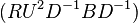# Rubik's cube group element with the smallest order

• Ahmed Abdullah

#### Ahmed Abdullah

Wikipedia says that largest order of any element of Rubik's cube group is 1260 [PLAIN]http://upload.wikimedia.org/math/e/1/c/e1cff178a2562422492a4140a38f93ff.png. [Broken] http://en.wikipedia.org/wiki/Rubik's_Cube_group
What about element of smallest order (except the identity element)? I'll appreciate any example with small order.

Last edited by a moderator:

What about element of smallest order (except the identity element)? I'll appreciate any
If you have an element G of order n, you can easily find an element with an order of any divisor d of n
$$G^{n/d}$$

•Ahmed Abdullah
Twisting any of the faces 180 degrees will have order 2, the smallest possible non-identity order.

 @willem2 beat me to it :D

Twisting any of the faces 180 degrees will have order 2, the smallest possible non-identity order.

Thank you. These are kind of uninteresting. Any interesting examples ... :)

If you have an element G of order n, you can easily find an element with an order of any divisor d of n
$$G^{n/d}$$
Is it easy to find? For example 10 divides the 1260 (order of). How I am going to find an element of order 10 from this ... ??

Is it easy to find? For example 10 divides the 1260 (order of). How I am going to find an element of order 10 from this ... ??
Apply ##(RU^2D^{-1}BD^{-1})## 1260/10 = 126 times. It will be good exercise for your wrists. :-)

•Ahmed Abdullah
Apply ##(RU^2D^{-1}BD^{-1})## 1260/10 = 126 times. It will be good exercise for your wrists. :)
I am not going to do that :)

Apply ##(RU^2D^{-1}BD^{-1})## 1260/10 = 126 times. It will be good exercise for your wrists. :)

But how I'll convert that position to L R U D notation?

But how I'll convert that position to L R U D notation?

•Ahmed Abdullah
Thank you very much. Now I know.

Thank you very much. Now I know.
This was actually a serious reply. Solvers that can find an optimum solution exist.
I downloaded the solver from http://kociemba.org/cube.htm and pasted in RU2D'BD' 126 times, and it immediately found D' R D2 R' D2 R F D2 F' D' R' D2
to generate the same pattern

•Ahmed Abdullah
This was actually a serious reply.

I know. And I found it extremely helpful. What I meant by "Now I know" is that, I can find out notation for any position by simply solving it by the cube solver. Actually it was the best thing that happened on the day. Thanks. :)